Win up to 100% scholarship on Aakash BYJU'S JEE/NEET courses with ABNAT Win up to 100% scholarship on Aakash BYJU'S JEE/NEET courses with ABNAT

# Complex Numbers

Complex numbers are defined as numbers of the form x+iy, where x and y are real numbers and i = √-1. For example, 3+2i, -2+i√3 are complex numbers. For a complex number z = x+iy, x is called the real part, denoted by Re z, and y is called the imaginary part, denoted by Im z. For example, if z = 3+2i, Re z = 3 and Im z = 2.

In this section, students will learn about complex numbers – definition, standard form, algebraic operations, conjugate, complex numbers’ polar form, Euler’s form and much more. A complex number is a combination of a real number and an imaginary number

## What Are Complex Numbers?

If x, y ∈ R, then an ordered pair (x, y) = x + iy is called a complex number. It is denoted by z, where x is the real part of Re(z) and y is the imaginary part or Im (z) of the complex number.

(i) If Re(z) = x = 0, then z is called a purely imaginary number.

(ii) If Im(z) = y = 0 then z is called a purely real number.

Note: The set of all possible ordered pairs is called a complex number set and is denoted by C.

### Integral Powers of an Iota

An imaginary number, i (iota), is defined as √-1 since i = √-1; we have i2 = –1, i3 = –i, and i4 = 1.

• To find the value of in (n > 4), first divide n by 4.

Let q be the quotient and r be the remainder.

n = 4q + r where o < r < 3

in = i4q + r = (i4)q . ir = (i)q . ir = ir

• The sum of four consecutive powers of i is zero.

in + in+1 + in + 2 + in + 3 = 0, n ∈ z

• 1/i = – i
• (1 + i)2 = 2i and (1 – i)2 = 2i
• √a . √b = √ab is valid only when at least one of a and b is non-negative.
• If both a and b both negative, then √a × √b = -√(|a|.|b|)
• √-a × √-b = -a

Illustration 1: Evaluate i201

Solution: 201 leaves the remainder as 1 when it is divided by 4; therefore, i201 = i1 = i.

Illustration 2: Evaluate 1 + (1+i) + (1+i)2 + (1+i)3

Solution: 1 + (1+i) + (1+i)2 + (1+i)3 = 1 + (1+i) + (2i) + (-2+2i)

= 1 + 1 + i + 2i  -2+2i = 5i

Illustration 3: [(1 + i)/√2]8n + [(1 – i)/√2]8n = ____.

Solution:

[(1 + i)/√2]8n + [(1 – i)/√2]8n = [{(1 + i)/√2}2]4n + [{(1 – i)/√2}2]4n

= (2i/2)4n + (-2i/2)4n = i4n + (-i)4n

= 1 + 1 = 2

Illustration 4: Evaluate: (i4n+1 – i4n-1)/2, n ε z.

Solution:

(i4n+1 – i4n – 1)/2 = (i4n . i – i4n . i-1)/2 = (i – i-1)/2 = (i + i)/2 = i.

### Algebraic Operations with Complex Numbers

1. Addition: (a + ib) + (c + id) = (a + c) + i(b + d)

2. Subtraction: (a + ib) – (c + id) = (ac) + i(b – d)

3. Multiplication: (a + ib) (c + id)

= (ac – bd) + i(ad + bc)

4. Reciprocal: If at least one of a, b is non-zero, then the reciprocal of a + bi is given by

1/(a+ib) = (a – ib)/[(a+ib) (a−ib)] = a/[a2 + b2] – i[b/(a2 + b2)]

5. Quotient: If at least one of c, d is non-zero, then the quotient of a + bi and c + di is given by

[(a+bi)/(c+di)] = [(a+ib) (c−id)]/[(c+id) (c−id)] = [(ac + bd) + i(bc – ad)]/[c2 + d2]

= [ac + bd]/[c2 + d2] + i[bc−ad]/[c2+d2 ]

### Conjugate of a Complex Number

Let = z = a + ib be a complex number. We define the conjugate of z, denoted by z¯, to be the complex number a – ib, that is, if z = a + ib, then z¯ = a – ib.

#### Properties of Conjugate of a Complex Number

1. $$\begin{array}{l}{{z}_{1}}={{z}_{2}}\Leftrightarrow {{\overline{z}}_{1}}={{\overline{z}}_{2}}[/latex 2. \(\begin{array}{l}\overline{(\bar{z})}=z\end{array}$$
3. $$\begin{array}{l}z+\overline{z}=2\,{Re}(z)\end{array}$$
4. $$\begin{array}{l}z-\overline{z}=2i\,{Im}\,(z)\end{array}$$
5. $$\begin{array}{l}z=\overline{z}\Leftrightarrow z\ \text{is purely real}\end{array}$$
6. $$\begin{array}{l}z+\overline{z}=0\Leftrightarrow z\ \text{is purely imaginary.}\end{array}$$
7. $$\begin{array}{l}z\overline{z}={{[Re\,(z)]}^{2}}+{{[Im(z)]}^{2}}\end{array}$$
8. $$\begin{array}{l}\overline{{{z}_{1}}+{{z}_{2}}}={{\overline{z}}_{1}}+{{\overline{z}}_{2}}\end{array}$$
9. $$\begin{array}{l}\overline{{{z}_{1}}-{{z}_{2}}}={{\overline{z}}_{1}}-{{\overline{z}}_{2}}\end{array}$$
10. $$\begin{array}{l}\overline{{{z}_{1}}{{z}_{2}}}={{\overline{z}}_{_{1}}}{{\overline{z}}_{_{2}}}\end{array}$$
11. $$\begin{array}{l}\overline{\left( \frac{{{z}_{1}}}{{{z}_{2}}} \right)}=\frac{{{\overline{z}}_{1}}}{{{z}_{2}}}\ \text{if}\ z_2 \ne 0\end{array}$$
12. If P(z) = a0 + a1 z + a2 z2 + …. + an zn
Where a0, a1, ….. an and z are complex number, then
$$\begin{array}{l}\overline{P(z)}={{\overline{a}}_{0}}+{{\overline{a}}_{1}}(\overline{z})+{{\overline{a}}_{2}}{{(\overline{z})}^{2}}+….+{{\overline{a}}_{n}}{{(\overline{z})}^{n}} = \overline{P}(\overline{z})\end{array}$$
Where
$$\begin{array}{l}\overline{P}(z)={{\overline{a}}_{0}}+{{\overline{a}}_{1}}z+{{\overline{a}}_{2}}{{z}^{2}}+….+{{\overline{a}}_{n}}{{z}^{n}}\end{array}$$
13. $$\begin{array}{l}\text{If}\ R(z) = \frac{P(z)}{Q(z)}\end{array}$$
where P (z) and Q (z) are polynomials in z, and Q(z) ≠ 0, then
$$\begin{array}{l}\overline{R\,(z)}=\frac{\overline{P}(\overline{z})}{\overline{Q}(\overline{z})}\end{array}$$

### Conjugates of Complex Numbers Video Lesson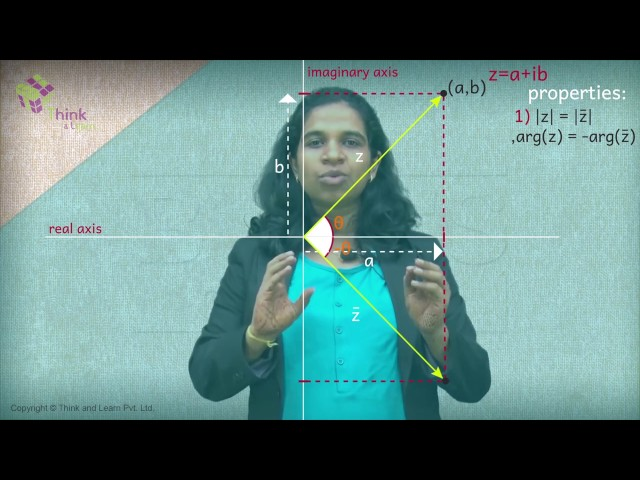## Modulus of a Complex Number

Let z = a + ib be a complex number. We define the modulus or the absolute value of z to be the real number √(a2 + b2) and denote it by |z|.

Note that |z| > 0 ∀ z ∈ C

### Properties of Modulus

If z is a complex number, then

(i) |z| = 0 ⇔ z = 0

(ii) |z| = |z¯| = |-z| = |-z¯|

(iii) – |z| ≤ Re (z) ≤ |z|

(iv) – |z| ≤ Im(z) ≤ |z|

(v) z z¯ = |z|2

If z1, z2 are two complex numbers, then

(vi) |z1 z2| = |z1|.|z2|

(vii) ∣z1/z2∣ = ∣z1/z2∣, if z2 ≠ 0

(viii) |z1 + z2|2 = |z1|2 + |z2|2 + z¯1 z2 + z1 z2  = |z1|2 + |z2|2 + 2Re (z12)

(ix) |z1+z2|2  + |z1|2 – |z2|2 – z¯­1 z2 – z12 = |z1|2 + |z2|2 – 2Re (z1 2)

(x) |z1+z2|2 + |z1 – z2|2 = 2(|z1|2 + |z2|2)

(xi) If a and b are real numbers and z1 and z2 are complex numbers, then |az1 + bz2 |2 + |bz1 – az2 |2 = (a2 + b2) (|z1|2 + |z2|2)

(xii) If z1, z2 ≠ 0, then |z1 + z2|2 = |z1|2 + |z2|2 ⇔z1 z2 is purely imaginary.

(xiii) Triangle Inequality. If z1 and z2 are two complex numbers, then |z1 + z2| < |z1| + |z2|. The equality holds if and only if z12 ≥ 0.

In general, |z+ z2+…+zn| < |z1| + |z2| +…..+ |zn| and the sign equality sign holds if and only if the ratio of any two non-zero terms is positive.

(xiv) |z1 – z2| ≤ |z1| + |z2|

(xv) ||z1| – |z2|| ≤ |z1| + |z2|

(xvi) |z1 – z2| ≥ ||z1| – |z2||

### Square Root of a Complex Number

Let z = x + iy then

$$\begin{array}{l}\sqrt{x+iy}=\left\{ \begin{matrix} \pm \left[ \sqrt{\frac{|z|+x}{2}}+i\sqrt{\frac{|z|-x}{2}} \right]if\,y\,>\,0 \\ \pm \left[ \sqrt{\frac{|z|+x}{2}}-i\sqrt{\frac{|z|-x}{2}} \right]if\,y\,<\,0 \\ \end{matrix} \right.\end{array}$$

Where

$$\begin{array}{l}|x| = \sqrt{{{x}^{2}}+{{y}^{2}}}\,\end{array}$$

NOTE:

(i)

$$\begin{array}{l}\sqrt{x+iy}+\sqrt{x-iy}=\sqrt{2|z|+2x}\end{array}$$

(ii)

$$\begin{array}{l}\sqrt{x+iy}-\sqrt{x-iy}=i\sqrt{2|z|-2x}\end{array}$$

(iii)

$$\begin{array}{l}\sqrt{i}=\pm \left( \frac{1+i}{\sqrt{2}} \right)\,and\,\sqrt{-i}=\pm \left( \frac{1-i}{\sqrt{2}} \right)\end{array}$$

### Modulus and Argument of a Complex Number

Let z = x + iy = (x, y) for all x, y R and

$$\begin{array}{l} i =\sqrt{-1}\end{array}$$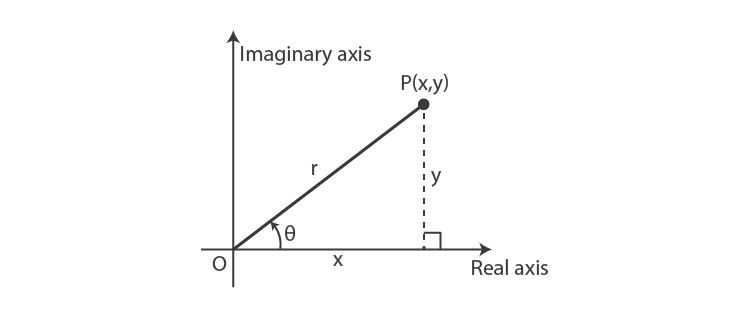The length OP is called the modulus of the complex number z denoted by |z|,

i.e., OP = r = |z|

$$\begin{array}{l}=\sqrt{({{x}^{2}}+{{y}^{2}})}\end{array}$$

And if (x, y) ≠ (0, 0), then θ is called the argument or amplitude of z,

$$\begin{array}{l}, i.e.,\ \theta = {{\tan }^{-1}}\left( \frac{y}{x} \right)\end{array}$$
[angle made by OP with positive X-axis]

or arg (z) =

$$\begin{array}{l}arg(z) = {{\tan }^{-1}}\left( \frac{y}{x} \right)\end{array}$$

Also, the argument of a complex number is not unique since if θ is a value of the argument, so also in 2nπ + θ, where n I. But usually, we take only that value for which

0 < θ < 2π. Any two arguments of a complex number differ by 2nπ.

The argument of z will be θ, π – θ, π + θ and 2π – θ according to the point z lies in I, II, III and IV quadrants, respectively, where

$$\begin{array}{l}\theta = {{\tan }^{-1}}\left| \frac{y}{x} \right|\end{array}$$
.

Illustration 5. Find the arguments of z1 = 5 + 5i, z2 = –4 + 4i, z3 = –3 – 3i and z4 = 2 – 2i, where

$$\begin{array}{l}i=\sqrt{-1}.\end{array}$$

Solution: Since z1, z2, z3 and z4 lies in I, II, III and IV quadrants, respectively, the arguments are given by

$$\begin{array}{l}arg(z_1) = {{\tan }^{-1}}\left( \frac{5}{5} \right)={{\tan }^{-1}}1=\pi /4\end{array}$$
$$\begin{array}{l}arg(z_2) =\pi -{{\tan }^{-1}}\left| \frac{4}{-4} \right|=\pi -{{\tan }^{-1}}1=\pi -\frac{\pi}{4}=\frac{3\pi }{4}\end{array}$$
$$\begin{array}{l}arg(z_3) =\pi -{{\tan }^{-1}}\left| \frac{-3}{-3} \right|=\pi +{{\tan }^{-1}}1=\pi +\frac{\pi}{4}=\frac{5\pi }{4}\end{array}$$

And

$$\begin{array}{l}arg(z_4) =2\pi -{{\tan }^{-1}}\left| \frac{-2}{2} \right|=2\pi +{{\tan }^{-1}}1=2\pi -\frac{\pi}{4}=\frac{7\pi }{4}\end{array}$$

#### Principal value of the argument

The value θ of the argument which satisfies the inequality –π < θ ≤ π is called the principal value of the argument.

If x = x + iy = ( x, y), x, y R and i = √(-1), then

$$\begin{array}{l}Arg(z) = {{\tan }^{-1}}\left( \frac{y}{x} \right)\end{array}$$
always gives the principal value. It depends on the quadrant in which the point (x, y) lies.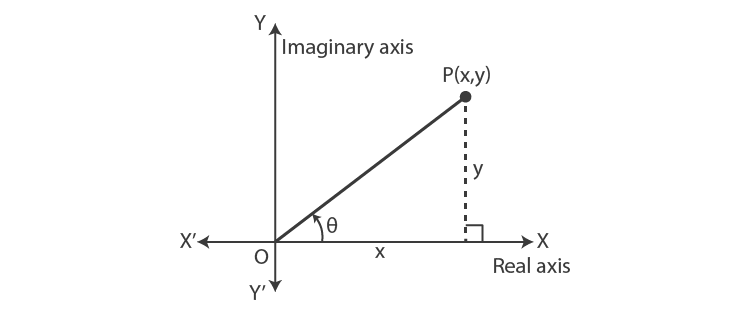(i) (x, y) first quadrant x > 0, y > 0.

The principal value of arg (z) = θ

$$\begin{array}{l}={{\tan }^{-1}}\left( \frac{y}{x} \right)\end{array}$$

It is an acute angle and positive.

(ii) (x, y) second quadrant x < 0, y > 0.

The principal value of arg (z) = θ

$$\begin{array}{l}=\pi -{{\tan }^{-1}}\left( \frac{y}{|x|}\right)\end{array}$$
. It is an obtuse angle and positive.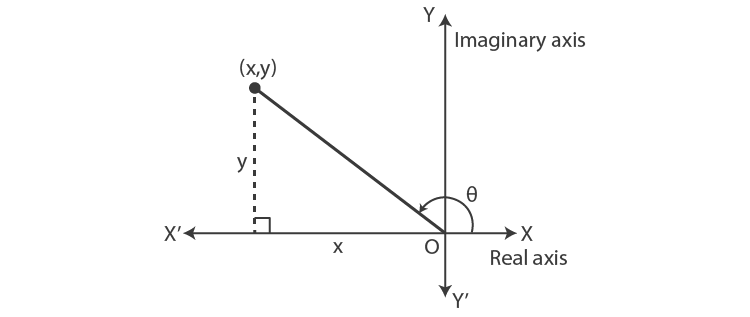(iii) (x, y) third quadrant x < 0, y < 0.

The principal value of arg (z) = θ

$$\begin{array}{l}=-\pi +{{\tan }^{-1}}\left( \frac{y}{x}\right)\end{array}$$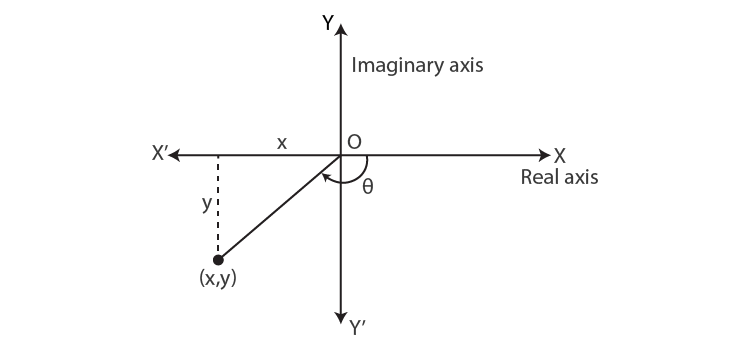It is an obtuse angle and negative.

(iv) (x, y) fourth quadrant x > 0, y < 0.

The principal value of arg (z) = θ

$$\begin{array}{l}= -{{\tan }^{-1}}\left( \frac{|y|}{x}\right)\end{array}$$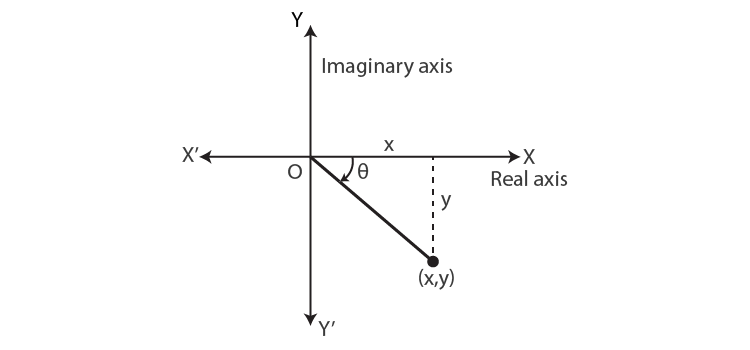It is an acute angle and negative.

### Polar Form of a Complex Number

We have, z = x + iy

$$\begin{array}{l}=\sqrt{{{x}^{2}}+{{y}^{2}}}\left[ \frac{2}{\sqrt{{{x}^{2}}+{{y}^{2}}}}+i\frac{x}{\sqrt{{{x}^{2}}+{{y}^{2}}}} \right]\end{array}$$

= |z| [cos θ + i sin θ]

Where |z| is the modulus of the complex number, i.e., the distance of z from the origin, and θ is the argument or amplitude of the complex number.

Here, we should take the principal value of θ. For general values of argument, z = r[cos(2nπ + θ)] (where n is an integer). This is a polar form of the complex number.

### Euler’s Form of a Complex Number

eiθ = cos θ + i sin θ

This form makes the study of complex numbers and their properties simple. Any complex number can be expressed as

z = x + iy (Cartesian form)

= |z| [cos θ + I sin θ] (polar form)

= |z| eiθ

#### De Moivre’s Theorem and Its Applications

(a) De Moivre’s theorem for integral index. If n is a integer, then (cos θ + i sin θ)n = cos (nθ) + i sin (nθ)

(b) De Moivre’s theorem for rational index. If n is a rational number, then the value of or one of the values of

(cos θ + i sin θ)n is cos (nθ) + i sin (nθ).

In fact, if n = p/q where p, q I, q > 0 and p,q have no factors in common, then (cos θ + i sin θ)n has q distinct values, one of which is cos (nθ) + i sin (nθ)

 Note: The values of (cos θ + I sin θ)p/q where p, q ∈ I, q ≠ 0, hcf (p, q) = 1 are given by $$\begin{array}{l}\cos \left[ \frac{p}{q}(2k\pi +\theta ) \right]+i\,sin\left[ \frac{p}{q}(2k\pi +\theta ) \right]\end{array}$$ Where k = 0, 1, 2, ….., q -1.

The nth Roots of Unity

By an nth root of unity, we mean any complex number z which satisfies the equation zn = 1 (1).

Since an equation of degree n has n roots, there are n values of z which satisfy the equation (1). To obtain these n values of z, we write 1 = cos (2kπ) + I sin (2kπ),

Where k ϵ I and

$$\begin{array}{l}\Rightarrow \,\,\,\,\,\,\,\,\,\,\,\,\,z=\cos \left( \frac{2k\pi }{n} \right)+i\sin \left( \frac{2k\pi }{n} \right)\end{array}$$
[using the De Moivre’s theorem]

Where, k = 0, 1, 2, …., n -1.

 Note: We may give any n consecutive integral values to k. For instance, in the case of 3, we may take -1, 0 and 1, and in the case of 4, we may take – 1, 0, 1 and 2 or -2, -1, 0 and 1.

Notation

$$\begin{array}{l}\omega =\cos \left( \frac{2\pi }{n} \right)+i\sin \left( \frac{2\pi }{n} \right)\end{array}$$

By using De Moivre’s theorem, we can write the nth roots of unity as 1, ω, ω2, …., ωn-1.

The sum of the roots of unity is zero.

We have

$$\begin{array}{l}1 + \omega + …. + \omega^{n-1} = \frac{1-{{\omega }^{n}}}{1-\omega }\end{array}$$

But ωn = 1 as ω is a nth root of unity.

$$\begin{array}{l}\therefore 1+\omega +…+{{\omega }^{n-1}}=0\end{array}$$

Also, note that

$$\begin{array}{l}\frac{1}{x-1}+\frac{1}{x-\omega }….+\frac{1}{x-{{\omega }^{n-1}}}=\frac{n{{x}^{n-1}}}{{{x}^{n}}-1}\end{array}$$

### nth Root of Unity Video LessonCube roots of unity

Cube roots of unity are given by 1, ω, ω2, where

$$\begin{array}{l}\omega =\cos \left( \frac{2\pi }{3} \right)+i\sin \left( \frac{2\pi }{3} \right)=\frac{-1+\sqrt{3i}}{2}and\,{{\omega }^{2}}=\frac{-1-\sqrt{3i}}{2}\end{array}$$
.

Some Results Involving Complex Cube Root of Unity (ω)

(i) ω3 = 1

(ii) 1 + ω + ω2 = 0

(iii) x3 – 1 = (x – 1) ( x – ω) (x – ω2)

(iv) ω and ω2 are roots of x2 + x + 1 = 0

(v) a3 – b3 = (a – b) (a – bω) (a – bω2)

(vi) a2 + b2 + c2 – bc – ca – ab

= (a + bω + cω2) (a + bω2 + cω)

(vii) a3 + b3 + c3 – 3abc

= (a + b + c) (a + bω + cω2) (a + bω2 + cω)

(viii) x3 + 1 = (x + 1) (x + ω) (x + ω2)

(ix) a3 + b3 = (a + b) (a + bω) (a + bω2)

(x) Cube roots of real number a are a1/3, a1/3ω, a1/3 ω2.

To obtain cube roots of a, we write x3 = a, as y3 = 1, where y = x/a1/3.

Solutions of y3 = 1 are 1, ω, ω2.

x = a1/3, a1/3 ω, a1/3 ω2.

### Cube Roots of Unity Video Lesson### Logarithm of a Complex Number

Loge(x + iy) = loge (|z|eiθ)

= loge |z| + loge eiθ

= loge |z| + iθ

$$\begin{array}{l}={{\log }_{e}}\sqrt{({{x}^{2}}+{{y}^{2}})}+i\arg (z)\end{array}$$
$$\begin{array}{l}\therefore {{\log }_{e}}(z)=lo{{g}_{e}}|z|+iarg(z)\end{array}$$

## Problems on Complex Numbers

Illustration 1: The number of solutions of

$$\begin{array}{l}{{z}^{3}}+\overline{z}=0\ \text{is}\end{array}$$

(a) 2 (b) 3 (c) 4 (d) 5

Ans. (d)

Solution

$$\begin{array}{l}{{z}^{3}}+\overline{z}=0\,\,\,\,\,\,\,\,\,\Rightarrow \,\,\,\,\,\,\,\,\,\,\,{{z}^{3}}=-\overline{z}\end{array}$$
$$\begin{array}{l}\Rightarrow \,\,\,\,\,\,\,\,\,\,\,|z{{|}^{3}}=|-\overline{z}|\,\,\,\,\,\,\,\Rightarrow \,\,\,\,\,\,|z{{|}^{3}}=|z|\end{array}$$
$$\begin{array}{l}|z|\,(|z|-1)\,(|z|+1)=0\end{array}$$

⇒ |z| = 0 or |z| = 1 [Since, |z| + 1 > 0]

If |z| = 0, then z = 0

$$\begin{array}{l}\text{If}\ |z|=1\ \text{we get};\ |z{{|}^{2}}=1\ \Rightarrow z\,\overline{z}=\,1\end{array}$$

Thus,

$$\begin{array}{l}{{z}^{3}}+\overline{z}=0\,\,\,\,\,\,\,\,\,\,\Rightarrow {{z}^{3}}+1/z=0\,\end{array}$$
$$\begin{array}{l}\Rightarrow \,\,{{z}^{4}}+1=0\end{array}$$

This equation has four non-zero and distinct roots. Therefore, the given equation has five roots.

TIP: It is unnecessary to find roots of z4 + 1 = 0

Illustration 2: If ω is an imaginary cube root of unity, then the value of the expression

2(1 + ω) (1 + ω2) + 3(2 + ω) (2 + ω2) + … + (n + 1) (n + ω) (n + ω2) is

$$\begin{array}{l}(a)\ \frac{1}{4}{{n}^{2}}{{(n+1)}^{2}}+n\end{array}$$
$$\begin{array}{l}(b)\ \frac{1}{4}{{n}^{2}}{{(n+1)}^{2}}-n\end{array}$$
$$\begin{array}{l}(c)\ \frac{1}{4}n{{(n+1)}^{2}}-n\end{array}$$

(d) none of these

Ans. (a)

Solution: The rth term of the given expression is

(r + 1) (r + ω) (r + ω2) = r3 + 1

The value of the given expression is

$$\begin{array}{l}\sum\limits_{r=1}^{n}{({{r}^{3}}+1)}=\frac{1}{4}{{n}^{2}}{{(n+1)}^{2}}+n\end{array}$$
.

Illustration 3: Find the real part of (1 – i)-i.

Sol: Let z = (1 – i)-i

Taking log on both sides, we have

$$\begin{array}{l}log\,z=-i\,lo{{g}_{e}}(1-i)\end{array}$$
$$\begin{array}{l}=-i{{\log }_{e}}\sqrt{2}\left( \cos \frac{\pi }{4}-i\sin \frac{\pi }{4} \right)\end{array}$$
$$\begin{array}{l}= -i{{\log }_{e}}(\sqrt{2}{{e}^{-i}}^{(\pi /4)})\end{array}$$
$$\begin{array}{l}=-i\left[ \frac{1}{2}{{\log }_{e}}2+{{\log }_{e}}^{-i\pi /4} \right]\end{array}$$
$$\begin{array}{l}= -i\left[ \frac{1}{2}{{\log }_{e}}2-\frac{i\pi }{4} \right]\end{array}$$
$$\begin{array}{l}= -\frac{i}{2}{{\log }_{e}}2-\frac{\pi }{4}\end{array}$$
$$\begin{array}{l}\Rightarrow \,\,\,\,\,\,\,\,\,\,\,z={{e}^{-\pi /4}}\,{{e}^{-i(log\,2)/2}}\end{array}$$
$$\begin{array}{l}\Rightarrow \,\,\,\,\,\,\,\,\,\,\,{Re}(z)={{e}^{-\pi /4}}\cos \left( \frac{1}{2}\log 2 \right)\end{array}$$

Illustration 4: If α, β and γ are the roots of x3 – 3x2 + 3x + 7 = 0 (ω is the cube roots of unity), find the value of

$$\begin{array}{l}\frac{\alpha -1}{\beta -1}+\frac{\beta -1}{\gamma -1}+\frac{\gamma -1}{\alpha -1}.\end{array}$$

Sol. We have, x3 – 3x2 + 3x + 7 = 0

$$\begin{array}{l}\Rightarrow {{(x-1)}^{3}}+8=0\end{array}$$
$$\begin{array}{l}\Rightarrow {{(x-1)}^{3}}+{{2}^{3}}=0\end{array}$$
$$\begin{array}{l}\Rightarrow (x-1+2)(x-1+2\omega )(x-1+2{{\omega }^{2}})=0\end{array}$$
$$\begin{array}{l}\Rightarrow (x+1)(x-1+2\omega )(x-1+2{{\omega }^{2}})=0\end{array}$$
$$\begin{array}{l}\therefore x=-1,1-2\omega ,1-2{{\omega }^{2}}\end{array}$$
,

$$\begin{array}{l}\Rightarrow \alpha =-1,\beta =1-2\omega ,\gamma=1-2{{\omega}^{2}}\end{array}$$

Then,

$$\begin{array}{l}\frac{\alpha -1}{\beta -1}+\frac{\beta -1}{\gamma -1}+\frac{\gamma -1}{\alpha -1}=\frac{-2}{-2\omega }+\frac{-2\omega }{-2{{\omega }^{2}}}+\frac{-2{{\omega }^{2}}}{-2}\end{array}$$
$$\begin{array}{l}=\frac{1}{\omega }+\frac{1}{\omega }+{{\omega }^{2}}={{\omega }^{2}}+{{\omega }^{2}}+{{\omega }^{2}}=3{{\omega }^{2}}\end{array}$$

Illustration 5: If z1 and z2 are 1 – i, -2 + 4i, respectively.

$$\begin{array}{l}\text{Find}\ {Im}\left( \frac{{{z}_{1}}{{z}_{2}}}{{{z}_{1}}} \right).\end{array}$$

Sol.

$$\begin{array}{l}\frac{{{z}_{1}}{{z}_{2}}}{{{z}_{1}}}=\frac{\left( 1-i \right)\left( -2+4i \right)}{1+i}=\frac{-2+2i+4i+4}{1+i}\end{array}$$
$$\begin{array}{l}=\frac{2+6i}{1+i}\times \frac{1-i}{1-i}=\frac{2+6i-2i+6}{2}=4+2i\end{array}$$
$$\begin{array}{l}\therefore {Im}\left( \frac{{{z}_{1}}{{z}_{2}}}{{{{\bar{z}}}_{1}}} \right)=2.\end{array}$$

Illustration 6: Find the square root of z = -7 – 24i.

Sol. Consider z0 = x + iy be a square root then;

$$\begin{array}{l}{{z}_{0}}^{2}=-7-24i.\end{array}$$

or

$$\begin{array}{l}-7-24i={{x}^{2}}-{{y}^{2}}+2ixy\end{array}$$

Equating real and imaginary parts, we get

$$\begin{array}{l}{{x}^{2}}-{{y}^{2}}=-7\end{array}$$

and 2xy = -24

$$\begin{array}{l}{{\left( {{x}^{2}}+{{y}^{2}} \right)}^{2}}={{\left( {{x}^{2}}-{{y}^{2}} \right)}^{2}}+4{{x}^{2}}{{y}^{2}}\end{array}$$
$$\begin{array}{l}={{\left( -7 \right)}^{2}}+{{\left( -24 \right)}^{2}}=625\end{array}$$
$$\begin{array}{l}\therefore {{x}^{2}}+{{y}^{2}}=25\end{array}$$

Solving (i) and (iii), we get,

$$\begin{array}{l}\left( x,y \right)=\left( 3,-4 \right);\left( -3,4 \right)\,by\,\left( ii \right)\end{array}$$
$$\begin{array}{l}\therefore {{z}_{0}}=\pm \left( 3-4i \right)\end{array}$$

Illustration 7: If n is a positive integer and ω be an imaginary cube root of unity, prove that

$$\begin{array}{l}1+{{\omega }^{n}}+{{\omega }^{2n}}=\left\{ \begin{matrix} 3,\,when\,n\,is\,a\,multiple\,of\,3 \\ 0,when\,n\,is\,not\,a\,multiple\,of\,3 \\ \end{matrix} \right.\end{array}$$

Sol. Case: I.

$$\begin{array}{l}n=3m;m\in I\end{array}$$
$$\begin{array}{l}\therefore 1+{{\omega }^{n}}+{{\omega }^{2n}}=1+{{\omega }^{3m}}+{{\omega }^{6m}}\end{array}$$
$$\begin{array}{l}=1+1+1\left[ Since,\;\;{{\omega }^{3}}=1 \right]=3\end{array}$$

Case: II.

$$\begin{array}{l}n=3m+1\,or\,3m+2;m\in I\end{array}$$

(a) Let n = 3m + 1

$$\begin{array}{l}\therefore L.H.S=1+{{\omega }^{3m+1}}+{{\omega }^{6m+2}}=1+\omega +{{\omega }^{2}}=0\end{array}$$

(b) Let n = 3m + 2

$$\begin{array}{l}1+{{\omega }^{3m+2}}+{{\omega }^{6m+4}}=1+{{\omega }^{2}}+{{\omega }^{4}}=1+{{\omega }^{2}}+\omega =0.\end{array}$$

Illustration 8: Show that

$$\begin{array}{l}\left| \frac{z-3}{z+3} \right|=2\end{array}$$
represents a circle.

Sol. Consider z = x + iy

$$\begin{array}{l}\therefore \left| \frac{z-3}{z+3} \right|=2\Rightarrow \left| \frac{x-3+iy}{x+3+iy} \right|=2\end{array}$$
$$\begin{array}{l}{{\left| x-3+iy \right|}^{2}}={{2}^{2}}{{\left| x+3+iy \right|}^{2}}\end{array}$$

or

$$\begin{array}{l}{{\left( x-3 \right)}^{2}}+{{y}^{2}}=4\left( {{\left( x+3 \right)}^{2}}+{{y}^{2}} \right)\end{array}$$
$$\begin{array}{l}\Rightarrow 3{{x}^{2}}+3{{y}^{2}}+30x+27=0\end{array}$$
,

which represents a circle.

Illustration 9: If |z1| = |z2| = …. = |zn| = 1, prove that

$$\begin{array}{l}\left| {{z}_{1}}{{z}_{2}}+…….+{{z}_{n}} \right|=\left| \frac{1}{{{z}_{1}}}+\frac{1}{{{z}_{2}}}+…….+\frac{1}{{{z}_{n}}} \right|\end{array}$$

Sol.

$$\begin{array}{l}\left| {{z}_{j}} \right|=1\Rightarrow {{z}_{j}}{{\bar{z}}_{j}}=1\forall j=1,……,n\end{array}$$
$$\begin{array}{l}\left( Since, \;z\bar{z}=\left| {{z}^{2}} \right| \right)\end{array}$$

L.H.S.

$$\begin{array}{l}\left| {{z}_{1}}{{z}_{2}}+…….+{{z}_{n}} \right|=\left| \frac{1}{{{z}_{1}}}+\frac{1}{{{z}_{2}}}+…….+\frac{1}{{{z}_{n}}} \right|=\end{array}$$
$$\begin{array}{l}\left| \overline{\frac{1}{{{z}_{1}}}+\frac{1}{{{z}_{2}}}+\frac{1}{{{z}_{3}}}…….+\frac{1}{{{z}_{n}}}} \right|\end{array}$$
$$\begin{array}{l}=\left| \overline{\frac{1}{{{z}_{1}}}+\frac{1}{{{z}_{2}}}+\frac{1}{{{z}_{3}}}…….+\frac{1}{{{z}_{n}}}} \right|=R.H.S.\end{array}$$

Illustration 10: If |z1 + z2| = |z1 – z2|, prove that arg(z1) – arg(z2) = odd multiple of π/2.

Sol. As we know

$$\begin{array}{l}\left| z \right|=z.\bar{z}.\end{array}$$
Apply this formula and consider
$$\begin{array}{l}z=r\left( \cos \theta +i\,sin\theta \right).\end{array}$$
$$\begin{array}{l}{{\left| {{z}_{1}}+{{z}_{2}} \right|}^{2}}={{\left| {{z}_{1}}-{{z}_{2}} \right|}^{2}}\end{array}$$
$$\begin{array}{l}\Rightarrow \left( {{z}_{1}}+{{z}_{2}} \right)\left( {{{\bar{z}}}_{1}}+{{{\bar{z}}}_{2}} \right)=\left( {{z}_{1}}-{{z}_{2}} \right)\left( {{{\bar{z}}}_{1}}-{{{\bar{z}}}_{2}} \right)\,\,or\end{array}$$
$$\begin{array}{l}{{z}_{1}}{{\bar{z}}_{1}}+{{z}_{2}}{{\bar{z}}_{2}}+{{z}_{2}}{{\bar{z}}_{1}}+{{z}_{1}}{{\bar{z}}_{2}}={{z}_{1}}{{\bar{z}}_{1}}+{{z}_{2}}{{\bar{z}}_{2}}-{{z}_{2}}{{\bar{z}}_{1}}-{{z}_{1}}{{\bar{z}}_{2}}\end{array}$$

or

$$\begin{array}{l}2\left( {{z}_{2}}{{{\bar{z}}}_{1}}+{{z}_{1}}{{{\bar{z}}}_{2}} \right)=0;{Re}\left( {{z}_{1}}{{{\bar{z}}}_{2}} \right)=0\end{array}$$

Let

$$\begin{array}{l}{{z}_{1}}={{r}_{1}}\left( \cos {{\theta }_{1}}+i\,\sin {{\theta }_{1}} \right)\,and\,{{z}_{2}}={{r}_{2}}\left( \cos {{\theta }_{2}}+i\,\sin {{\theta }_{2}} \right);\end{array}$$

then

$$\begin{array}{l}{{z}_{1}}{{\bar{z}}_{2}}={{r}_{1}}{{r}_{2}}\left( \cos \left( {{\theta }_{1}}-{{\theta }_{2}} \right)+i\,\sin \left( {{\theta }_{1}}-{{\theta }_{2}} \right) \right)\end{array}$$
$$\begin{array}{l}\therefore \cos \left( {{\theta }_{1}}-{{\theta }_{2}} \right)=0\left( as\,{Re}\left( {{z}_{1}}{{{\bar{z}}}_{2}} \right)=0 \right)\end{array}$$
,

$$\begin{array}{l}{{\theta }_{1}}-{{\theta }_{2}}=\text{odd multiple of} \ \frac{\pi }{2}.\end{array}$$

Illustration 11: If |z – 1| < 3, prove that |iz + 3 – 5i| < 8.

Sol: Here, we have to reduce iz + 3 – 5i as the sum of two complex numbers containing z – 1 because we have to use

|z – 1| < 3.

|iz + 3 – 5i| = |iz – i + 3 – 4i| = |3 – 4i + i (z – 1) | < |3 – 4i| + |i (z – 1 )|

(by triangle inequality) < 5 + (1 . 3) =5+3= 8

Illustration 12: If (1 + x)n = a0 + a1x + a2x2+ ….+ anxn, then show that

$$\begin{array}{l}(a)\ {{a}_{0}}-{{a}_{2}}+{{a}_{4}}+….={{2}^{\frac{n}{2}}}\cos \frac{n\pi }{4}\end{array}$$
$$\begin{array}{l}(b)\ {{a}_{1}}-{{a}_{3}}+{{a}_{5}}+….={{2}^{\frac{n}{2}}}\sin \frac{n\pi }{4}\end{array}$$

Sol: Simply put x = i in the given expansion, and then by using the formula

z = r (cos θ + i sin θ) and (cos θ + i sin θ)n

= cos nθ + i sin nθ, we can solve this problem.

Put x = i in the given expansion

(1 + i)n = a0 + a1i + a2i2 + ….+ anin.

$$\begin{array}{l}{{\left[ \sqrt{2}\left( \cos \frac{\pi }{4}+i\sin \frac{\pi }{4} \right) \right]}^{n}}\end{array}$$
= (a0 – a2 + a4 – …) + i (a1 – a3 + a5 – …)

$$\begin{array}{l}{{2}^{n/2}}\left( \cos \frac{n\pi }{4}+i\sin \frac{n\pi }{4} \right)\end{array}$$
= (a0 – a2 + a4 + …. ) + i (a1 – a3 + a5 + …)

Equating real and imaginary parts,

$$\begin{array}{l}{{2}^{\frac{n}{2}}}{{\cos }^{\frac{n\pi }{4}}}={{a}_{0}}-{{a}_{2}}+{{a}_{4}}+…..\end{array}$$
$$\begin{array}{l}{{2}^{\frac{n}{2}}}{{\sin }^{\frac{n\pi }{4}}}={{a}_{1}}-{{a}_{3}}+{{a}_{5}}+…..\end{array}$$

Therefore, (a) and (b) are proved.

Illustration 13: Solve the equation

$$\begin{array}{l}{{z}^{n-1}}=\overline{z}:n\in N\end{array}$$

Sol: Apply modulus on both sides.

$$\begin{array}{l}{{z}^{n-1}}=\overline{z};\,\,\,\,\,\,\,\,|z{{|}^{n-1}}=|\overline{z}|=|z|\end{array}$$
$$\begin{array}{l}\therefore |z|=0\,or\,|z|\,=1\,\,If\,|z|=0\,then\,z\,=\,0,\end{array}$$
,

$$\begin{array}{l}Let\left| z \right|=1;\text{then},\text{ }{{z}^{n}}=z\overline{z}=1\end{array}$$
$$\begin{array}{l}\therefore z=\cos \frac{2m\pi }{n}+\sin \frac{2m\pi }{n}:m=0,\,1,\,…..,\,n-1\end{array}$$

Illustration 14: If z = x + iy and

$$\begin{array}{l}\omega =\frac{1-iz}{z-i}\end{array}$$

with |ω| = 1, show that z lies on the real axis.

Sol: Substitute value of ω in |ω| = 1

$$\begin{array}{l}\left| \omega \right|=\left| \frac{1-iz}{z-i} \right|=1\Rightarrow |1-iz|=|z-i|\end{array}$$
or, |1 – ix + y| = |x + i(y – 1)|

or, (1 + y)2 + x2 = x2 + (y – 1)2 or, 4y = 0

Hence, z lies on the real axis.

Illustration 15: If a complex number z lies in the interior or on the boundary of a circle of radius as 3 and centre at (0, –4), then the greatest and least values of |z + 1| are,

$$\begin{array}{l}(a)\ 3+\sqrt{17},\sqrt{17}-3\end{array}$$

(b) 6, 1

$$\begin{array}{l}(c)\ \sqrt{17},1\end{array}$$

(d) 3, 1

Sol:  The greatest and least value of |z + 1| means a maximum and minimum distance of the circle from the point (–1, 0). In a circle, the greatest and least distance of it from any point is along the normal.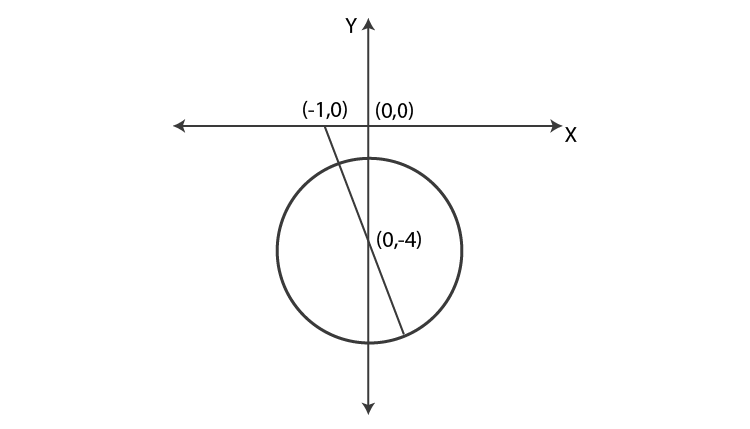$$\begin{array}{l}\therefore \text{Greatest distance} =\,3+\sqrt{{{1}^{2}}+{{4}^{2}}}=3+\sqrt{17}\end{array}$$
$$\begin{array}{l}\text{Least distance} =\sqrt{{{1}^{2}}+{{4}^{2}}}-3=\sqrt{17}-3\end{array}$$

Illustration 16: Find the equation of the circle for which arg

$$\begin{array}{l}\left( \frac{z-6-2i}{z-2-2i} \right)=\pi /4.\end{array}$$

Sol:

$$\begin{array}{l}\arg \left( \frac{z-6-2i}{z-2-2i} \right)=\pi /4\end{array}$$

represent a major arc of the circle, of which the line joining (6, 2)) and (2, 2) is a chord that subtends an angle π/4 at the circumference.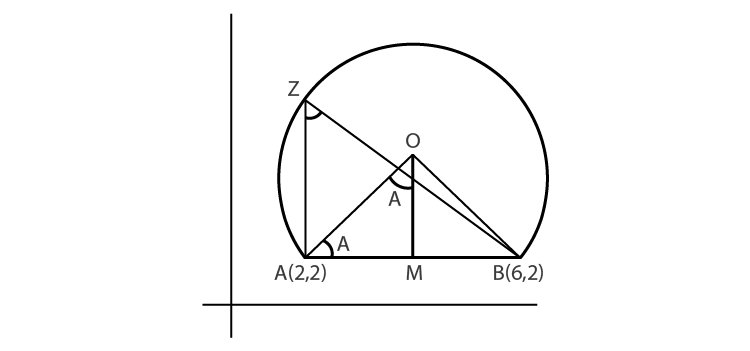Clearly, AB is parallel to the real (x) axis, M is the mid-point, M ≡ (4, 2), OM = AM = 2

$$\begin{array}{l}\therefore O=(4,4)\ \text{and}\ O{{A}^{2}}=O{{M}^{2}}+A{{M}^{2}}=2\sqrt{2}\end{array}$$

The equation of the required circle is

$$\begin{array}{l}|z-4-4i|=2\sqrt{2}\end{array}$$

Illustration 17: if |z| > 3, prove that the least value of

$$\begin{array}{l}\left| z+\frac{1}{z} \right|\,is\,\frac{8}{3}\end{array}$$
.

Sol: Here,

$$\begin{array}{l}\left| z+\frac{1}{z} \right|\,\underline{>}\,|z|-\frac{1}{|z|}\end{array}$$

Now, |z| > 3

$$\begin{array}{l}\therefore \frac{1}{|z|}\underline{<}\frac{1}{3}\,or\,-\frac{1}{|z|}\underline{>}-\frac{1}{3}….(i)\end{array}$$

$$\begin{array}{l}|z|-\frac{1}{|z|}\underline{>}3-\frac{1}{3}=\frac{8}{3}….(ii)\end{array}$$

Hence, from (i) and (ii), we get

$$\begin{array}{l}\left| z+\frac{1}{z} \right|\underline{>}\frac{8}{3}\end{array}$$

∴ The least value is 8/3.

Illustration 18: If z1, z2, z3 are non-zero complex numbers such that

$$\begin{array}{l}{{z}_{1}}+{{z}_{2}}+{{z}_{3}}=0\, and\,z_{1}^{-1}+z_{2}^{-1}+z_{3}^{-1}=0\end{array}$$
, then prove that the given points are the vertices of an equilateral triangle. Also, show that

|z1| = |z2| = |z3|.

Sol: Use algebra to solve this problem.

Given z1 + z2 + z3 = 0, and from 2nd relation z2z3 + z3z1 + z1z2 = 0

$$\begin{array}{l}\therefore {{z}_{2}}{{z}_{3}}=-{{z}_{1}}({{z}_{2}}+{{z}_{3}})=-{{z}_{1}}(-{{z}_{1}})=z_{1}^{2}\end{array}$$
$$\begin{array}{l}\therefore {{z}_{1}}^{3}={{z}_{1}}{{z}_{2}}{{z}_{3}}=z{{{}_{2}}^{3}}={{z}_{3}}^{3}\end{array}$$
$$\begin{array}{l}\therefore |{{z}_{1}}{{|}^{3}}=|{{z}_{2}}{{|}^{3}}=|{{z}_{3}}{{|}^{3}}\end{array}$$

Above shows that the distance of origin from A, B, and C is the sample.

Origin is circumcentre, but z1 + z2 + z3 = 0.

This implies that the centroid is also at the origin, so the triangle must be equilateral.

Illustration 19: For constant c > 1, find all complex numbers z satisfying the equation z + c |z + 1| + i = 0.

Sol: Solve this by putting z = x + iy.

Let z = x + iy

The equation z + c |z + 1| + i = 0 becomes

$$\begin{array}{l}x+iy+c\sqrt{{{(x+1)}^{2}}+{{y}^{2}}}+i=0\end{array}$$
$$\begin{array}{l}or\,x+c\sqrt{{{(x+1)}^{2}}+{{y}^{2}}}+i(y+1)=0\end{array}$$

Equating real and imaginary parts, we get

y + 1 = 0 y = –1 ….(i)

and

$$\begin{array}{l}x+c\sqrt{{{(x+1)}^{2}}+{{y}^{2}}}=0:x<0….(ii)\end{array}$$

By solving (i) and (ii), we get

$$\begin{array}{l}x+c\sqrt{{{(x+1)}^{2}}+1}=0\,or\,{{x}^{2}}={{c}^{2}}[{{(x+1)}^{2}}+1]\end{array}$$

Or (c2 – 1)x2 + 2c2x + 2c2 = 0

If c = 1, then x = –1. Let c > 1, then,

$$\begin{array}{l}x=\frac{-2{{c}^{2}}\pm \sqrt{4{{c}^{4}}-8{{c}^{2}}({{c}^{2}}-1)}}{2({{c}^{2}}-1)}=\frac{-{{c}^{2}}\pm c\sqrt{2-{{c}^{2}}}}{{{c}^{2}}-1}\end{array}$$

As x is real and c > 1, we have:

$$\begin{array}{l}1<c\le \sqrt{2}\end{array}$$

(Thus, for √2, there is no solution). Since both values of x satisfy (ii), both values are admissible.

Illustration 20: Find the sixth roots of z = 64i.

Sol:

$$\begin{array}{l}\text{Here},\ i=\cos \frac{\pi }{2}+i\sin \frac{\pi }{2}\end{array}$$
and sixth root of z

i.e., zr = z1/6.

$$\begin{array}{l}z=64\left( \cos \frac{\pi }{2}+i\sin \frac{\pi }{2} \right)\end{array}$$
$$\begin{array}{l}{{z}_{r}}={{z}^{1/6}}\end{array}$$
$$\begin{array}{l}=2\left( \cos \frac{2r\pi +\frac{\pi }{2}}{6}+\sin \frac{2r\pi +\frac{\pi }{2}}{6} \right)\end{array}$$

Where, r = 0, 1, 2, 3, 4, 5

The roots z0, z1, z3, z4, z5 are given by

$$\begin{array}{l}{{z}_{0}}=2\left( \cos \frac{\pi }{12}+i\sin \frac{\pi }{12} \right)\end{array}$$
$$\begin{array}{l}{{z}_{1}}=2\left( \cos \frac{5\pi }{12}+i\sin \frac{5\pi }{12} \right)\end{array}$$
$$\begin{array}{l}{{z}_{2}}=2\left( \cos \frac{9\pi }{12}+i\sin \frac{9\pi }{12} \right)\end{array}$$
$$\begin{array}{l}{{z}_{3}}=2\left( \cos \frac{13\pi }{12}+i\sin \frac{13\pi }{12} \right)=-2\left( \cos \frac{\pi }{12}+i\sin \frac{\pi }{12} \right)\end{array}$$
$$\begin{array}{l}{{z}_{4}}=2\left( \cos \frac{17\pi }{12}+i\sin \frac{17\pi }{12} \right)=-2\left( \cos \frac{5\pi }{12}+i\sin \frac{5\pi }{12} \right)\end{array}$$
$$\begin{array}{l}{{z}_{5}}=2\left( \cos \frac{21\pi }{12}+i\sin \frac{21\pi }{12} \right)=-2\left( \cos \frac{9\pi }{12}+i\sin \frac{9\pi }{12} \right)\end{array}$$

Illustration 21: Let three vertices, A, B, and C (taken in clockwise order) of an isosceles right-angled triangle with a right angle at C, be affixes of complex numbers z1, z2, z3, respectively. Show that (z1 – z2)2 = 2(z1 – z3) (z3 – z2).

Sol: Here,

$$\begin{array}{l}\frac{{{z}_{2}}-{{z}_{3}}}{{{z}_{1}}-{{z}_{3}}}={{e}^{-i\pi /2}}.\end{array}$$

Therefore, solve it using the algebra method.

Given CB = CA and angle ∠C = π/2,

$$\begin{array}{l}\frac{{{z}_{2}}-{{z}_{3}}}{{{z}_{1}}-{{z}_{3}}}={{e}^{-i\pi /2}}\end{array}$$
or (z3 – z2)2 = i2(z1 – z3)2

(z3 – z2)2 = -(z1 – z3)2

Or

$$\begin{array}{l}z_{3}^{2}+z_{2}^{2}-2{{z}_{2}}{{z}_{3}}+z_{1}^{2}+z_{3}^{2}-2{{z}_{1}}{{z}_{3}}=0\end{array}$$

Add and subtract 2z1z2, and we get

$$\begin{array}{l}z_{1}^{2}+z_{2}^{2}-2{{z}_{1}}{{z}_{2}}+2z_{3}^{2}+2{{z}_{2}}{{z}_{3}}-2{{z}_{1}}{{z}_{3}}+2{{z}_{1}}{{z}_{2}}=0,\,or\end{array}$$
$$\begin{array}{l}{{({{z}_{1}}-{{z}_{2}})}^{2}}+2({{z}_{3}}-{{z}_{1}})({{z}_{3}}-{{z}_{2}})=0,\,or\,{{({{z}_{1}}-{{z}_{2}})}^{2}}=2({{z}_{1}}-{{z}_{3}})({{z}_{3}}-{{z}_{2}}).\end{array}$$

Illustration 22: If A, B, C be the angles of a triangle, then

$$\begin{array}{l}\text{prove that}\ \left| \begin{matrix} {{e}^{2iA}} & {{e}^{-iC}} & {{e}^{-iB}} \\ {{e}^{-iC}} & {{e}^{2iB}} & {{e}^{-iA}} \\ {{e}^{-iB}} & {{e}^{-iA}} & {{e}^{2iC}} \\ \end{matrix} \right|\ \text{is purely real}.\end{array}$$

Sol: Here, A + B + C = π; therefore, epi = cos π + i sin π = –1. And by using the properties of matrices, we can solve this problem.

e-pi = –1 …. (i)

ei(B+C) = ei(π–A) = epi e–iA = –e–iA

ei(B+C)= –eiA …. (ii)

Take eiA, eiB and eiC common from R1, R2 and R3, respectively. Δ = ei(A+B+c)

$$\begin{array}{l}\,\left| \begin{matrix} {{e}^{iA}} & {{e}^{-i(A+C)}} & {{e}^{-i(A+B)}} \\ {{e}^{-i(B+C)}} & {{e}^{iB}} & {{e}^{-i(B+A)}} \\ {{e}^{-i(B+C)}} & {{e}^{-i(C+A)}} & {{e}^{iC}} \\ \end{matrix} \right|=-1\left| \begin{matrix} {{e}^{iA}} & -{{e}^{iB}} & -{{e}^{i}} \\ -{{e}^{iA}} & {{e}^{iB}} & -{{e}^{iC}} \\ -{{e}^{iA}} & -{{e}^{iB}} & {{e}^{iC}} \\ \end{matrix} \right|,by\,(ii)\end{array}$$

Take eiA, eiB and eiC common from C1, C2 and C3 and again put ei(A+B+C)=e = –1

$$\begin{array}{l}\therefore \Delta =(-1)(-1)\left| \begin{matrix} 1 & -1 & -1 \\ -1 & 1 & -1 \\ -1 & -1 & 1 \\ \end{matrix} \right|\end{array}$$

Now, make two zeros and expand Δ = –4, which is purely real.

Illustration 23: Show that all the roots of the equation

$$\begin{array}{l}{{z}^{n}}cos{{q}_{0}}+{{z}^{n-1}}cos\text{ }{{q}_{1}}+{{z}^{n-2}}cos\,{{q}_{2}}+\ldots ..+z\,cos\text{ }{{q}_{n-1}}+cos\text{ }{{q}_{n}}=2\end{array}$$

lie outside the circle |z| = 1/2 where q0, q1 etc. are real.

Sol: By using triangle inequality,

$$\begin{array}{l}\left| {{z}^{n}}cos\text{ }{{q}_{0}}+{{z}^{n-1}}cos\text{ }{{q}_{1}}+{{z}^{n-2}}\text{ }cos\text{ }{{q}_{2}}\text{ }+\text{ }\ldots .\text{ }+\text{ }z\text{ }cos\text{ }{{q}_{n-1}}+cos\text{ }{{q}_{n}} \right|=2\end{array}$$

By the triangle inequality,

$$\begin{array}{l}2 = |{{z}^{n}}cos{{\theta }_{n}}+{{2}^{n-1}}cos\,{{q}_{1}}+{{2}^{n-1}}\,\cos \,{{q}_{2}}+…+z\cos \,{{q}_{n-1}}+\cos \,{{q}_{n}}|\le |{{z}^{n}}cos{{q}_{n}}|+|{{z}^{n-1}}cos\,{{q}_{1}}|\end{array}$$
+

$$\begin{array}{l}|{{z}^{n-2}}cos\,{{q}_{2}}|+….++|zcos\,{{q}_{n-1}}|+|cos\,{{q}_{n}}|=|{{z}_{n}}||cos\,{{q}_{n}}|+|{{z}^{n-1}}||cos\,{{q}_{n}}|+…+|z|cos\,{{q}_{n-1}}|+\end{array}$$
$$\begin{array}{l}|cos\,{{q}_{n}}|\,\le \,|z{{|}^{n}}+|z{{|}^{n-1}}+….+|z|+1\end{array}$$
$$\begin{array}{l}\left( Since,\;\,|cos\,{{q}_{1}}|\le 1\,and\,|{{z}^{n-1}}|=|z{{|}^{n+1}} \right)\end{array}$$
$$\begin{array}{l}=\frac{1-|z{{|}^{n+1}}}{1-|z|}<\frac{1}{1-|z|}\end{array}$$
$$\begin{array}{l}\therefore 2<\frac{1}{1-|z|}\end{array}$$
$$\begin{array}{l}sol\,1-|z|is\,positive\,and\,1-|z|\,<\frac{1}{2}\end{array}$$
$$\begin{array}{l}\therefore \,|z|>1-\frac{1}{2}=\frac{1}{2}\end{array}$$

∴ All z satisfying (i) lie outside the circle, |z| = 1/2.

Illustration 24: If z + (1/z) = 2 cos θ, prove that

$$\begin{array}{l}\left| \frac{{{z}^{2n}}-1}{{{z}^{2n}}+1} \right|=|tan\,nq|\end{array}$$

Sol: By using the formula of roots of a quadratic equation, we can solve this problem.

Here, z + 1/z = 2 cos θ

$$\begin{array}{l}\therefore {{z}^{2}}-2\cos \,\theta .\,z+1=0\end{array}$$
$$\begin{array}{l}\therefore z=\frac{2\cos \theta \pm \sqrt{4{{\cos }^{2}}\theta -4}}{2}=\cos \theta \pm i\sin \theta\end{array}$$

Taking positive the sign,

$$\begin{array}{l}\therefore \frac{1}{z}={{(cos\,\theta \,+\,i\,sin\,\theta )}^{-1}}=cos\,\theta \,-\,i\,sin\,\theta\end{array}$$
$$\begin{array}{l}\therefore \frac{{{z}^{2n}}-1}{{{z}^{2n}}+1}=\frac{{{z}^{n}}-\frac{1}{{{z}^{n}}}}{{{z}^{n}}+\frac{1}{{{z}^{n}}}}=\frac{{{(cos\theta +isin\theta )}^{n}}-{{(cos\theta -isin\theta )}^{n}}}{{{(cos\theta +isin\theta )}^{n}}+{{(cos\theta -isin\theta )}^{n}}}\end{array}$$
$$\begin{array}{l}=\frac{\cos n\theta +i\sin \theta -(cosn\theta -isin\theta )}{\cos n\theta +i\sin \theta +(cosn\theta -isinn\theta }=\frac{2i\,\sin n\theta }{2\cos n\theta }=i\tan n\theta\end{array}$$
.

Taking the negative sign,

Similarly, we get

$$\begin{array}{l}\frac{{{z}^{2n}}-1}{{{z}^{2n}}+1}=\frac{-2i\sin \,n\theta }{2\cos n\theta }=-i\tan \,n\theta\end{array}$$
$$\begin{array}{l}\therefore \left| \frac{{{z}^{2n}}-1}{{{z}^{2n}}+1} \right|=|\pm i\,tan\,nq|=|tan\,nq|,\end{array}$$

Illustration 25: Find the complex number z, which satisfies the condition |z – 2 + 2i| = 1 and has the least absolute value.

Sol: Here, z – 2 + 2i = cos θ + i sin θ; therefore, by obtaining the modulus of z, we can solve the above problem.

|z – 2 + 2i| = 1

$$\begin{array}{l}\Rightarrow \,z-2+2i=\cos \,\theta +i\,\sin \,\theta\end{array}$$

Where θ is some real numbers.

$$\begin{array}{l}\Rightarrow \,z=(2+cos\theta )+(sin\theta -2)i\end{array}$$
$$\begin{array}{l}\Rightarrow \,|z|={{[{{(2+cos\,\theta )}^{2}}+{{(sin\theta -2)}^{2}}]}^{1/2}}\end{array}$$
$$\begin{array}{l}={{[8+co{{s}^{2}}\theta +si{{n}^{2}}\theta +4(cos\theta -\sin \theta )]}^{1/2}}\end{array}$$
$$\begin{array}{l}=\,{{\left[ 9+4\sqrt{2}\cos \left( \theta +\frac{\pi }{4} \right) \right]}^{1/2}}\end{array}$$

|z| will be least if cos (θ + π/4) is least, that is, if cos (θ + π/4) = -1 or θ = 3π/4.

Thus, the least value of |z| is

$$\begin{array}{l}{{\left( 9-4\sqrt{2} \right)}^{1/2}}for\,z=\left( 2-\frac{1}{\sqrt{2}} \right)+i\left( \frac{1}{\sqrt{2}}-2 \right)\end{array}$$

Illustration 26: The area of the triangle whose vertices are represented by the complex numbers 0, z,

$$\begin{array}{l}z{e}^{i\alpha},(0<\alpha <\pi) \text{equals}\end{array}$$

Sol:

Vertices are

$$\begin{array}{l}0=0+i0,z=x+iy \text \ and \ z{{e}^{i\alpha}}\\=(x+iy)\,\,(\cos \alpha +i\sin \alpha)=(x\cos \alpha -y\sin \alpha )+i(y\cos \alpha +x\sin \alpha )\\ Area =\frac{1}{2}\,\left| \,\begin{matrix} 0 & 0 & 1 \\ x & y & 1 \\ (x\cos \alpha -y\sin \alpha )\,\,\, & (y\cos \alpha +x\sin \alpha )\,\, & 1 \\ \end{matrix}\, \right|\\=\frac{1}{2}[xy\cos \alpha +{{x}^{2}}\sin \alpha -xy\cos \alpha +{{y}^{2}}\sin \alpha ]\\=\frac{1}{2}\sin \alpha ({{x}^{2}}+{{y}^{2}})\\=\frac{1}{2}|z{{|}^{2}}\sin \alpha [\,\,|z|=\sqrt{{{x}^{2}}+{{y}^{2}}}].\\\end{array}$$

Illustration 27: The complex numbers z = x + iy which satisfy the equation

$$\begin{array}{l}\left| \frac{z-5i}{z+5i} \right|=1\ \text{lie on}\end{array}$$

Sol:

$$\begin{array}{l}\left| \frac{z-5i}{z+5i} \right|=1\\ \left| \frac{x+i(y-5)}{x+i(y+5)} \right|=1 \\ |x+i(y-5)|\,=\,|x+i(y+5)|, \left(\left| \frac{{{z}_{1}}}{{{z}_{2}}} \right|=\frac{|{{z}_{1}}|}{|{{z}_{2}}|} \right) \\ {{x}^{2}}+25-10y+{{y}^{2}}={{y}^{2}}+{{x}^{2}}+25+10y \\ 20y=0 \\ y=0\\\end{array}$$

Illustration 28: The region of the complex plane for which

$$\begin{array}{l}\left| \frac{z-a}{z+\overline{a}} \right|=1\,\,[R(a)\ne 0]\ \text{is}\end{array}$$

Sol:

We have

$$\begin{array}{l}\left| \frac{z-a}{z+\bar{a}} \right|=1 \\ |z-a|\,=\,|z+\overline{a}| \\ |z-a{{|}^{2}}=|z+\overline{a}{{|}^{2}} \\ (z-a)(\overline{z-a})=(z+\overline{a})(\overline{z+\overline{a}}) \\ (z-a)(\overline{z}-\overline{a})=(z+\overline{a})(\overline{z}+a) \\ z\overline{z}-z\overline{a}-a\overline{z}+a\overline{a}=z\overline{z}+za+\overline{a}\overline{z}+\overline{a}a \\ za+z\overline{a}+\overline{a}\overline{z}+a\overline{z}=0\, \Rightarrow (a+\overline{a})(z+\overline{z})=0 \\ z+\overline{z}=0\,\,(a+\overline{a}=2 {Re}(a)\ne 0) \\ 2 {Re}(z)=0\end{array}$$

2x = 0

x = 0, which is the equation of y-axis.

Illustration 29:

$$\begin{array}{l}\frac{3+2 i \sin \theta}{1-2 i \sin \theta}\ \text{will be purely imaginary, if}\ \theta\ \text{equals}\\ (1) 2 \mathrm{n} \pi \pm \frac{\pi}{3}\\ (2) \mathrm{n} \pi+\frac{\pi}{3}\\ (3) \mathrm{n} \pi \pm \frac{\pi}{3}\\ (4) \text { None of these}\end{array}$$

Sol:

$$\begin{array}{l}\frac{3+2 i \sin \theta}{1-2 i \sin \theta}\ \text{will be purely imaginary, if the real part vanishes}\\ \begin{array}{l} \frac{3-4 \sin ^{2} \theta}{1+4 \sin ^{2} \theta}=0 \\ \Rightarrow 3-4 \sin ^{2} \theta=0 \quad \text { (only if } \theta \text { be real) } \\ \Rightarrow \sin \theta=\pm \frac{\sqrt{3}}{2}=\sin \left(\pm \frac{\pi}{3}\right) \\ \Rightarrow \theta=n \pi+(-1)^{n}\left(\pm \frac{\pi}{3}\right)=n \pi \pm \frac{\pi}{3} \end{array}\\ Answer: \end{array}$$

Illustration 30:

$$\begin{array}{l}\text{If}\ \frac{\tan \theta-i\left(\sin \frac{\theta}{2}+\cos \frac{\theta}{2}\right)}{1+2 i \sin \frac{\theta}{2}}\ \text{is purely imaginary, then general value of}\ \theta\ \text{is} -\\ (1) \mathrm{n} \pi+\frac{\pi}{4}\\ (2) 2 n \pi+\frac{\pi}{4}\\ (3) \mathrm{n} \pi+\frac{\pi}{2}\\ (4) 2 n \pi+\frac{\pi}{2}\end{array}$$

Sol:

Multiply above and below by the conjugate of the denominator and put the real part equal to zero.

$$\begin{array}{l}\begin{array}{l} =\frac{\tan \theta-i\left(\sin \frac{\theta}{2}+\cos \frac{\theta}{2}\right)}{1+2 i \sin \frac{\theta}{2}} \times \frac{1-2 i \sin \frac{\theta}{2}}{1-2 i \sin \frac{\theta}{2}} \\ =\frac{\tan \theta-2 \sin \frac{\theta}{2}\left(\sin \frac{\theta}{2}+\cos \frac{\theta}{2}\right)-i\left(\sin \frac{\theta}{2}+\cos \frac{\theta}{2}+2 \tan \theta \sin \frac{\theta}{2}\right)}{1+4 \sin ^{2} \frac{\theta}{2}} \\ \therefore \tan \theta-2 \sin \frac{\theta}{2}\left(\sin \frac{\theta}{2}+\cos \frac{\theta}{2}\right)=0 \\ \Rightarrow \frac{\sin \theta}{\cos \theta}-(1-\cos \theta)-\sin \theta=0 \\ \Rightarrow \sin \theta\left(\frac{1-\cos \theta}{\cos \theta}\right)-(1-\cos \theta)=0 \\ \Rightarrow(1-\cos \theta)(\tan \theta-1)=0 \\ \cos \theta=1 \Rightarrow \theta=2 n \pi \text { and } \tan \theta=1 \Rightarrow \theta=n \pi+\frac{\pi}{4} \end{array}\\ Answer: \end{array}$$

Illustration 31:

$$\begin{array}{l}\sum_{\mathrm{k}=1}^{6}\left(\sin \frac{2 \pi \mathrm{k}}{7}-\mathrm{i} \cos \frac{2 \pi \mathrm{k}}{7}\right) \text { is equal to}\\ (1) -1\\ (2) 0\\ (3) i\\ (4) -i\end{array}$$

Sol:

$$\begin{array}{l}\text { Expression } =-i \sum_{k=1}^{6}\left(\cos \frac{2 \pi k}{7}+i \sin \frac{2 \pi k}{7}\right) \\ =-i \sum_{k=1}^{6} e^{\frac{2 \pi k}{7} i} \\ =-i\left[e^{\frac{2 \pi}{7} i}+e^{\frac{4 \pi}{7} i}+\ldots \ldots e^{\frac{12 \pi}{7}}\right] \\ =-i e^{\frac{2 \pi}{7}} \frac{\left(1-e^{\frac{12 \pi}{7} i}\right)}{\left(1-e^{\frac{2 \pi}{7} i}\right)}=-i \frac{e^{\frac{2 \pi}{7}}-e^{2 \pi i}}{1-e^{\frac{2 \pi}{7}}}=i\left(\frac{1-e^{\frac{2 \pi}{7}}}{1-e^{\frac{2 \pi}{7}}}\right) \\ =i\\ Answer: \end{array}$$

## Complex Numbers Rapid Revision for JEE## Complex Numbers Questions for JEE Main### Quadratic Equations and Complex Numbers Important JEE Main Questions### Complex Numbers – Top 12 Most Important and Expected JEE Questions### Also, Check:

Geometry of Complex Numbers

Complex Numbers Class 11

## Complex Numbers – Important Topics## Complex Numbers – Important QuestionsQ1

### What do you mean by a complex number?

A complex number is defined as a number of the form z = p+iq, where p and q are real numbers, and i = √-1. For example, 7-2i and 1+i are complex numbers.

Q2

### How to do the multiplication of two complex numbers?

Let z1 = a+ib, z2 = c+id be two complex numbers. Then, z1z2 = (a+ib)(c+id) = (ac – bd) + i(bc + ad). Always note that i2 = -1.

Q3

### What do you mean by the real part and imaginary part of a complex number?

In a complex number, z = a+ib, a is called the real part and b is called the imaginary part. The real part is represented by Re z, and the imaginary part is represented by Im z.

Test your knowledge on Complex Numbers